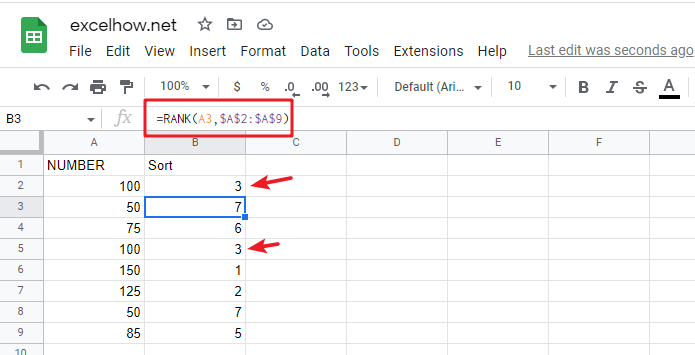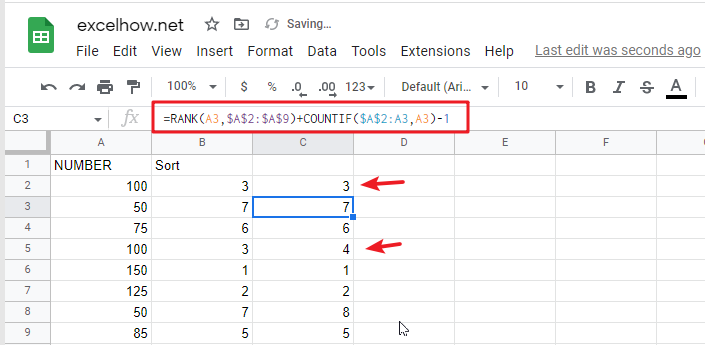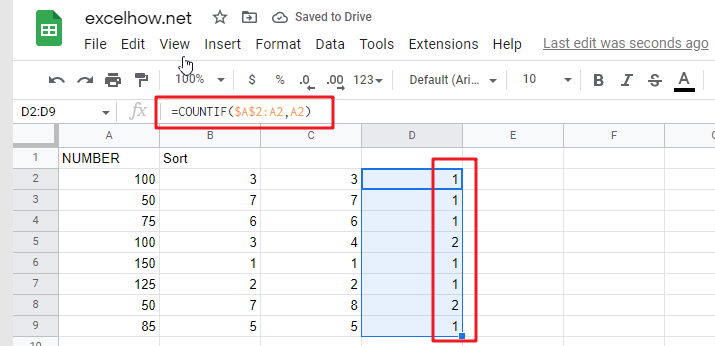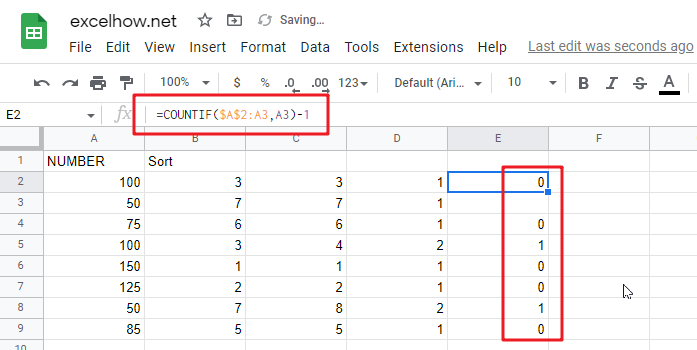# Rank Numeric Values with Duplicate in Google Sheets

Suppose you have an urgent task of ranking a list with duplicate values, what would be the first approach you would think of? Is there a quick and easy way to do this task in Google Sheets? This article will talk about how to rank a given list that contains duplicate values by using the RANK formula in google Sheets.

The Rank function is a built-in function of google sheets that can be used to rank the values in a set; in other words, if the given list includes duplicate values, then the rank number will also be duplicate. See the following example.From the above screenshot, you can see that the cell range A2:A9 includes 2 of the same number 100, which is ranked as 3 in the whole list, and there is no value ranked as 4 in the sorting column, because the value ranked 4 has been occupied by 3.

If we want to avoid this problem (4 should be assigned to the second 100), when we encounter a duplicate number in a set of numbers, instead of assigning it the previously used rank number, we will assign it a new rank number that follows exactly the previous rank number.

If you want to avoid the above problem of the ranked values being occupied, then you can use the following formula based on the Google Sheets RANK and COUNTIF functions：

`=RANK(A2,\$A\$2:\$A\$9)+COUNTIF(\$A\$2:A2,A2)-1`Then you need to press the shortcut to allow other cells in the sorted column to apply the formula as well. CTRL+Enter

## Let’s See How This Formula Works:

As mentioned above, if you just use the Google Sheets Rank function to sort a given list of values, and as soon as it contains duplicate values, then the duplicate ranking values will be displayed in the sort results column.

When sorting a list of values, the following 2 key points need to be cleared：

• Whether the sorted result is in ascending or descending order
• Whether the sorted result can contain duplicate numbers or must be unique

### RANK EXPLANATION

You can simply think that the RANK function in google sheets is used to rank a given list of values in ascending or descending order.

Syntax:

`=RANK(number, reference, [order])`
• number – Need to determine the number of rankings
• reference– a set of numbers for ranking
• order – optional; ascending (1) or descending order (0)

You can specify whether the RANK function sorts in ascending or descending order as you need. For example, for a running race , you can use ascending order to sort, and for sales result , you can use descending order to sort. For the RANK function, the default sorting option is ascending.

### COUNTIF EXPLANATION

The COUNTIF function in google sheets is used to count the number of cells that meet a given criteria.

Syntax:

`=COUNTIF(range, criteria)`

### FORMULA EXPLANATION

=RANK(A2,\$A\$2:\$A\$9)

In this example, the numbers are arranged in a fixed range. To avoid the range being adjusted when copying the formula, so we add \$ to lock the range. This formula works well if there are no duplicate numbers, and the RANK function will assign 1 to the largest number and 2 to the second largest number according to the rule, and so on.

Unfortunately, the given list of values have duplicate values, in order to avoid duplicate ranking values, you must use the RANK function and COUNTIF function to build a new google sheets formula together.=COUNTIF(\$A\$2:A2,A2)

When copying the formula downward, the given range \$A\$2:A2 is extended, the starting cell A2 is locked, and the ending cell is the cell where the RANK function is currently working. Therefore, If the COUNTIF function encounters a duplicate value when counting, it will return the number of times that value has been repeated.

• If number only appears once, COUNTIFreturns 1 as current row is included in selected range;
• If number appears twice, COUNTIF returns 2, and so on;=COUNTIF(\$A\$2:A2,A2)-1

Since the number appears at least once in the selected range, for example, COUNTIF(\$A\$2:A2,A2) returns 1, we use the subtraction operation “-1” to offset the value returned by COUNTIF.